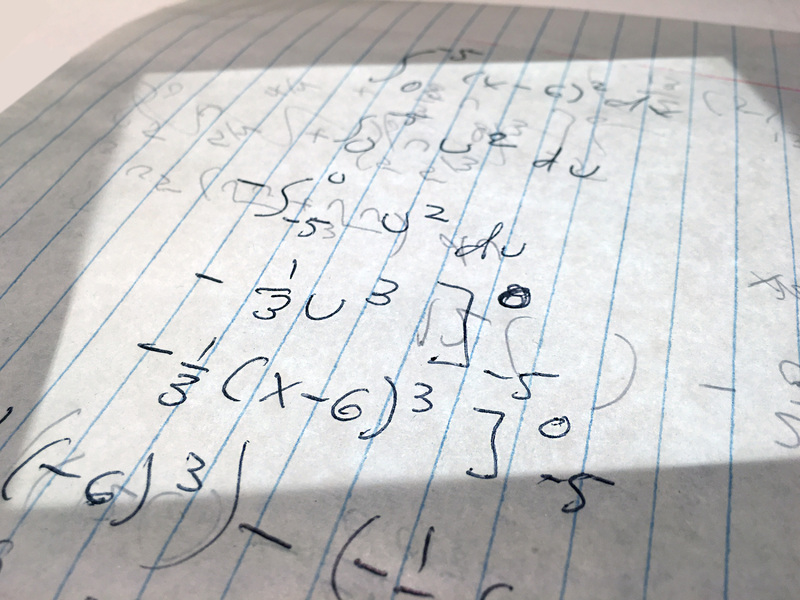# Eleven crazy learnings from the Java 11 certification: division by zero (5/11)

2 minute read ◦ posted on 26 Jul 2022 by .

In the summer of 2021, I got my Java 11 certification. I expected it to be quite a breeze, because I’d been a Java developer for 14 years and surely I should have seen it all by now, right? Turned out I was very wrong. I came across lots of things that I didn’t even know were possible with Java. In this weekly blog series I will go through 11 of these ‘crazy learnings’ that surprised me the most, even as an experienced developer. This week we’ll get mathematical as we focus on dividing by zero in Java.

## Dividing by zero has no meaning

What happens if you divide a number by zero? You probably learned all about this mathematical quirk in your middle or high school mathematics class. In ordinary arithmetic, an expression that consists of a division by zero has no meaning, which is why we were taught that such an expression evaluates to undefined. This simple rule is reflected in the Java language by the `java.lang.ArithmeticException`. An exception of this type is thrown each time you try to divide by zero. At least, that was my belief until I got my Java 11 certification.

## Dividing ints

Sure enough, when we would divide any Integer in Java by zero…

``````public class DivisionByZero {
public static void main(String[] args) {
System.out.println(divide(42, 0));
}

public static int divide(int dividend, int divisor) {
return dividend / divisor;
}
}
``````

…we would get an ArithmeticException thrown at runtime:

`Exception in thread "main" java.lang.ArithmeticException: / by zero`

## Dividing floats and doubles

OK, so we get an ArithmeticException when dividing an integer by zero. And I knew already that similar behaviour is displayed when dividing `BigDecimal`s. So when I got a practice exam question on the use case demonstrated above, but with floats instead of ints, I didn’t hesitate and marked the answer that said “RuntimeException is thrown”. Turned out I was wrong!

Now, if we would overload the `divide` method for floats and doubles, we would quickly discover why.

``````public class DivisionByZero {
public static void main(String[] args) {
//System.out.println(divide(42, 0));
System.out.println(divide(42f, 0f));
System.out.println(divide(42.0, 0.0));
}

public static int divide(int dividend, int divisor) {
return dividend / divisor;
}

public static float divide(float dividend, float divisor) {
return dividend / divisor;
}

public static double divide(double dividend, double divisor) {
return dividend / divisor;
}
}
``````

This program returns:

`Infinity`

`Infinity`

So apparently floats and doubles can hold infinity values, as opposed to integers and BigDecimals.

If we turn to the Java Language Specification, it turns out I could have known about this behaviour had I taken the time to read the specification through. Because this is what it has to say on the subject:

Division of a nonzero finite value by a zero results in a signed infinity.

and:

Division of a zero by a zero results in NaN.

(This behaviour is in accordance with the IEEE Standard for Floating-Point Arithmetic (IEEE 754).)Image from PxHere

## Other blog posts in this series

Did you miss a blog post in this series? Here’s a list of all posts that have been published so far:

Tags:

Updated: